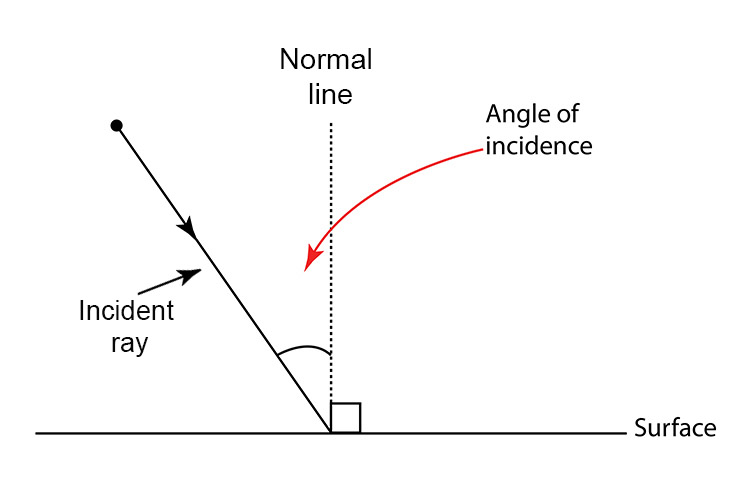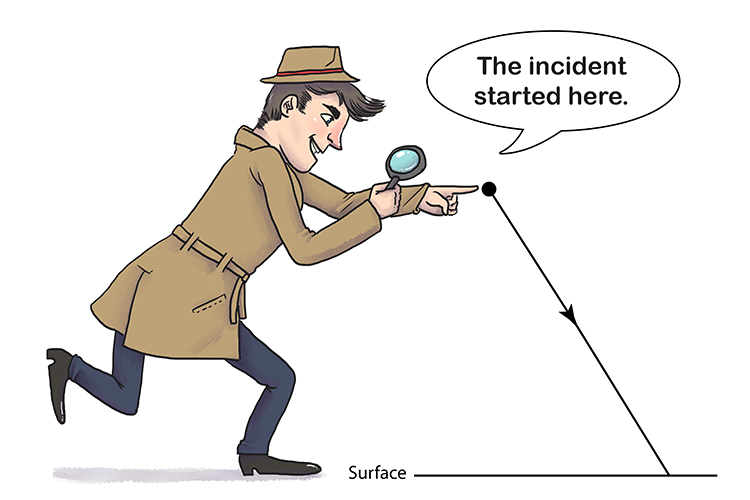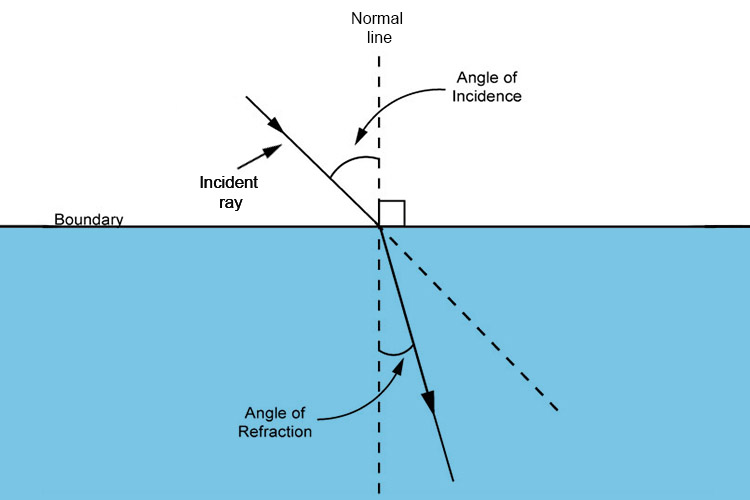# Angle of incidence and angle of refraction

The angle of incidence is the angle between a ray of light which hits the surface and the normal line. The ray of light is called the incident ray.The following should help you to remember which is the incident ray:Remember the "incident" started here. This will help you remember that the ray of light has to start when an incident occurs.

The angle of refraction is the angle between the refracted ray and the normal line.The following examples show how the angle of refraction differs from the angle of incidence:

• Angle of incidence in air 40° – angle of refraction in water 29°
• Angle of incidence in air 40° – angle of refraction in glass 25°